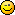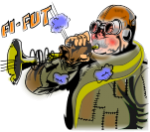Author Topic: Rounding numbers!  (Read 2181 times)Ozden79

• Mr. Polyvector
•• Posts: 121Rounding numbers!
« on: 2010-Jan-29 »
In some cases, I need to round a number to closest upper and some cases into closest lower integer value. I only see INTEGER command which I think only rounds to closest lower integer number. Is there any such command in GLBasic or do I need to implement my own?

Thanks,

Ãƒâ€“zdenQuentin

• Prof. Inline
•• Posts: 915Re: Rounding numbers!
« Reply #1 on: 2010-Jan-29 »
if you want to round it business like, you can try this (I think it was already ask somewhere though)

Code: (glbasic) [Select]
PRINT round(5.434344), 0, 0
PRINT round(5.66344), 0, 20
SHOWSCREEN
KEYWAIT

// ------------------------------------------------------------- //
// ---  ROUND%  ---
// ------------------------------------------------------------- //
FUNCTION round%: p_num
RETURN INTEGER(p_num + .5)
ENDFUNCTION // ROUND%Kitty Hello

• code monkey
• Prof. Inline
••• Posts: 10816
• here on my island the sea says 'hello'Re: Rounding numbers!
« Reply #2 on: 2010-Feb-01 »
bad for negative numbersYou could round like this:
Code: (glbasic) [Select]
FUNCTION Round: number, prec
LOCAL mulf = MAX(POW(10., prec), 1e-12)
number = number *mulf
LOCAL ret
IF number<0
RETURN INTEGER(number-.5)/mulf
ENDIF
RETURN INTEGER(number+.5)/mulf

ENDFUNCTION

But usually you only want rounded numbers for output. Then use FORMAT\$().Qedo

• Dr. Type
••• Posts: 355
• to program what I have do how should programming?Re: Rounding numbers!
« Reply #3 on: 2020-Apr-05 »
Good for positive and negative numbers

FUNCTION round%: p_num
RETURN INTEGER(p_num+(SGN(p_num)*0.5))
ENDFUNCTION // ROUND%